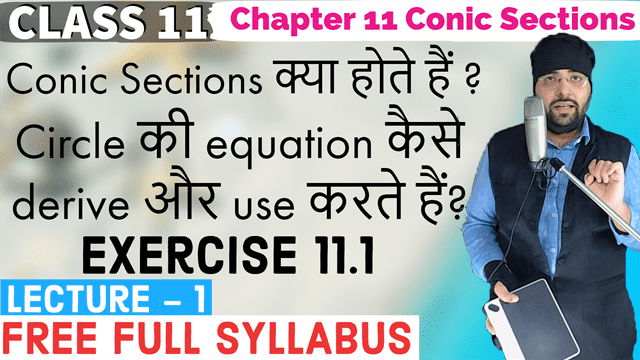# Exercise 11.1 Chapter 11 Conic Sections Class 11 Maths## Topics/Questions discussed in this video:

In this lecture I have discussed ncert solutions for Exercise 11.1 of Chapter 11 Conic Sections Class 11 Maths.

00:00:05 How to study Conic Sections in Class 11

00:05:31 Introduction to Circle, Parabola, Ellipse and Hyperbola

00:07:31 Animation to understand why they are called conic sections

00:09:51 How to derive equation of circle
In each of the following Exercises 1 to 5, find the equation of the circle with

00:13:51 NCERT Solutions Exercise 11.1 Question 1 centre (0,2) and radius 2

00:16:41 NCERT Solutions Exercise 11.1 Question 2 centre (–2,3) and radius 4

00:18:31 NCERT Solutions Exercise 11.1 Question 3 centre \left ( \frac{1}{2}, \frac{1}{4} \right ) and radius \frac{1}{12}

00:21:41 NCERT Solutions Exercise 11.1 Question 4 centre (1,1) and radius \sqrt{2}

00:22:52 NCERT Solutions Exercise 11.1 Question 5 centre (–a, –b) and radius \sqrt{a^2-b^2}
In each of the following Exercises 6 to 9, find the centre and radius of the circles.

00:25:32 NCERT Solutions Exercise 11.1 Question 6 (x+5)^2+(y-3)^2=36

00:26:52 NCERT Solutions Exercise 11.1 Question 7 x^2+y^2-4x-8y-45=0

00:31:02 NCERT Solutions Exercise 11.1 Question 8 x^2+y^2-8x+10y-12=0

00:33:22 NCERT Solutions Exercise 11.1 Question 9 2x^2+2y^2-x=0

00:36:02 NCERT Solutions Exercise 11.1 Question 15 Does the point (–2.5, 3.5) lie inside, outside or on the circle x^2+ y^2 = 25?

00:42:42 NCERT Solutions Exercise 11.1 Question 14 Find the equation of a circle with centre (2,2) and passes through the point (4,5).

00:45:42 NCERT Solutions Exercise 11.1 Question 12 Find the equation of the circle with radius 5 whose centre lies on x-axis and passes through the point (2,3).

00:51:42 NCERT Solutions Exercise 11.1 Question 13 Find the equation of the circle passing through (0,0) and making intercepts a and b on the coordinate axes.

00:59:42 NCERT Solutions Exercise 11.1 Question 10 Find the equation of the circle passing through the points (4,1) and (6,5) and whose centre is on the line 4x + y = 16.

01:08:32 NCERT Solutions Exercise 11.1 Question 11 Find the equation of the circle passing through the points (2,3) and (–1,1) and whose centre is on the line x – 3y – 11 = 0.

#### Tags## Agam Sir

Teacher

Ashish Kumar a.k.a Agam Sir has vast experience of 12+ years of teaching Mathematics and Physics. He has skills (academic and vocational) that go with working alongside people, especially young people, their parent(s) and the institutes that works with him. He always seek to be an agent of positive change and progression, a mentor/ trainer/ educator for learners/students, seeing that their needs are ideally met.